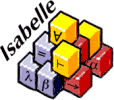Hello there! I recently came across Proof Assistants and was really intrigued by them. While these do not hold mainstream attention as of now, most proof assistants are being used to help solve various research problems in mathematics and computer science. Due to their appreciable benefits, I decided to write this post to lightly touch upon two of them - Coq and Isabelle. Finally, we will be writing a very simple proof in both the languages to see them in action.

## What are Proof Assistants?

Proof Assistants are software that allow you to translate mathematical proofs into formal proofs which can be then verified by a computer. Therefore, their work extends towards verification of mathematical theorems and an attempt to formalize mathematics. As these proof assistants have compilers which read and accept only a particular style of inputs given to them, it could be argued that they are programming languages.

## Coq & Isabelle

Coq and Isabelle are examples of proof assistants. Without making this explanation too complicated (since I am no expert myself), Coq uses a set of languages (which we will come to later) to understand a proof and verify it. The idea behind Coq and even Isabelle is to be able to break the proof down into some subgoals that each has to be proved. As a result, if each of these subgoals is proved, the end result is that the proof has been verified.

### Installing Coq

I’m assuming you are using some form of Linux, otherwise you may head over here to download it.

• On Ubuntu, running sudo apt-get install coq will take care of everything

### Installing Isabelle

Again, if you’re not using Linux, head over here.

• First run wget https://isabelle.in.tum.de/dist/Isabelle2017_app.tar.gz
• Then, tar -xzf Isabelle2017_app.tar.gz
• To start up the IDE, run Isabelle2017/Isabelle2017. It will take some time to start up the first time!

## Basics of Coq

It would be a good idea to get an understanding of how Coq works. There are three types of language sets that Coq uses to verify proofs:

• Gallina: This is used to write down what needs to be proved.
• Tactics: This aids the writing of the proofs and the commands are always lower-case.
• Vernacular: This part of Coq handles definitions and they keywords used always start with an upper-case letter.

It is also important to note that in Coq every statement ends with a . just like the ; is used to terminate statements in C/C++.

### A general reference for Tactics

This is just a list of very few tactics employed by Coq with some simple explanations behind their functions. Don’t worry if you don’t understand any of these now– It’s just to get an idea of what tactics look like and their functions.

Tactic Function
intros Introduce terms into the proof
simpl Simplifies the subgoal
destruct Destructs the current term into subgoals
induction Destructs the current term by adding a predefined inductive hypothesis
rewrite Rewrites one subgoal with another
reflexivity Solves a subgoal where LHS = RHS
assumption Solves the subgoal if a defined hypothesis exists
contradiction Solves the subgoal if a contradictory hypothesis (not an equality) exists

### A general reference for Vernacular commands

Command Function
Theorem Starts the theorem and is followed by the theorem name
Lemma Same as Theorem
Remark Same as Theorem
Fact Same as Theorem
Corollary Same as Theorem
Proposition Same as Theorem
Qed Hence, proved

## Basics of Isabelle

While by no means have we studied Coq in-depth, Isabelle is an even tougher nut to crack. I will describe the core syntax of an Isabelle proof below and then we will directly see the Isabelle proof for the theorem we seek to prove today. Again, do not panic if you don’t understand anything. These are more of a reference than anything else.

### Core syntax

 proof proof method statement qed proof (contd.) by method method (simp . . .) or (blast . . .) or (rule . . .) statement fix variables (^) statement (contd.) assume prop (=>) statement (contd.) from fact (have-show) prop proof statement (contd.) next (separates subgoals) prop name “formula” fact name name-of-fact “formula”

### Other important abbreviations

 this the previous proposition proved/assumed then from this thus then show hence then have

## The Theorem to prove

The theorem we seek to prove is really simple. It’s the associativity of addition for natural numbers. What this translates into mathematically is (for A, B and C as natural numbers),

I do not think this warrants much explanation, and we will jump into the proofs straight away.

## Proof in Coq

The first task ahead of ourselves is to define the ‘addition’ operation itself as Coq doesn’t define this on its own. The best way to learn to do this is by looking at the way we would actually do it. For two natural numbers (nat)f and s as inputs to the addition operation, we write the addition operation as a recursive function. The upper case ‘S’ denotes the successor to whatever is placed after it. Therefore, our addition operation is defined as follows:

We see that our definition is correct as CoqIDE says the following: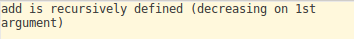Next, we will go on and prove our theorem first by defining our proof using Gallina and then proving it. We will cover each line of code and see what subgoals remain to be proved for our problem.

We can see that for all a,b and c natural numbers, 1 subgoal exists: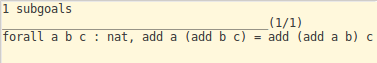We can see that Coq has been introduced to the 3 arguments using the intros tactic: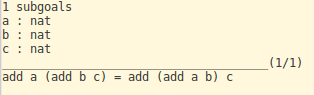Next, we use induction on ‘a’ to break down the problem into two subgoals: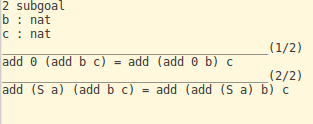Then we use simpl to simplify the expression at hand by using the definition of addition that we had introduced early on.

The simplified subgoals can be seen now: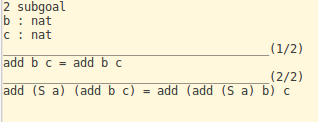Next we use reflexivity to reduce the already solved subgoal and get our first proven inductive hypothesis IHa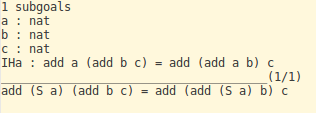Then we simplify again to solve through the second subgoal: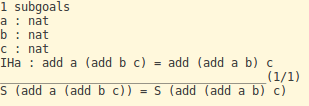What this gives us is just our first inductive hypothesis. As a result, we can simply rewrite our current subgoal as IHa and then finally use reflexivity to prove our proposed associativity theorem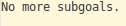Finally we write Qed (look at Vernacular commands) and prove our theorem!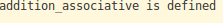Here is the complete program:

## Proof in Isabelle

The Isabelle theorem follows the same ideas that we incorporated into our Coq proof, but the syntax is a little different. An important thing to remember is that the name of the file (for example, here - “example.thy”) should be the same as what is followed by theory in the first line.

Next, we define the proof for addition here as well. Again, we define it recursively and the concept behind it is exactly the same, with the exception of the syntax.

Now we start our proof. Here we need to define cases for the induction on ‘a’ and proceed with the proof using known logical tools such as reflexivity and simplify. It will be a good exercise to read through the proof and try and figure out that it’s just like the Coq proof. Also, I wouldn’t blame you if you liked Coq more. To me (someone with very little experience in formal logic and proof verification) it seems more intuitive and simpler than Isabelle.

I would recommend that you go through the each line and see the subgoals remaining in Isabelle. If everything went by correctly you should see something like this: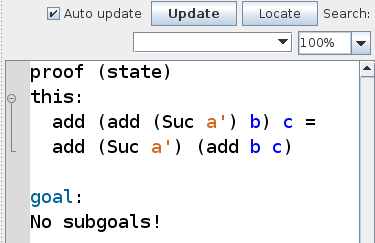Here is the complete program:

## Conclusion

With this post, I was hopefully able to interest you enough in the usefulness of proof assistants. Hopefully, you would like to learn more. There’s some excellent Coq (and Isabelle) tutorials out there that you can look up if you’re interested - here, here and here. See you next time!